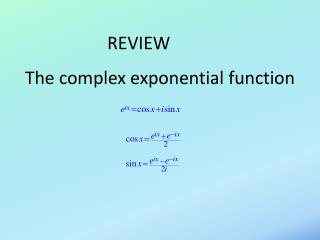DownloadDownload PresentationThe complex exponential function

# The complex exponential function

Download Presentation## The complex exponential function

- - - - - - - - - - - - - - - - - - - - - - - - - - - E N D - - - - - - - - - - - - - - - - - - - - - - - - - - -
##### Presentation Transcript

1. Hyperbolic functions

2. Differential eigenvalue problems

3. Partial derivatives Increment: x part y part

4. Totalderivatives x part y part t part

5. The material derivative:derivative “following the motion” x part y part t part v u

6. Multivariate Calculus 2:partial integrationseparation of variablesFourier methods

7. Partial differential equations Algebraicequation: involves functions; solutions are numbers. Ordinary differential equation (ODE): involves total derivatives; solutions are univariate functions. Partial differential equation (PDE): involves partial derivatives; solutions are multivariate functions.

8. Notation

9. Classification

10. Order =order of highest derivative with respect to any variable.

12. Partial integration

13. Partial integration

14. Partial integration

15. Partial integration

16. Solution by separation of variables Try to reduce PDE to two (or more) ODEs.

17. The Plan

18. Example 1

19. Example 1

20. Example 1

21. Example 1

22. Thermal diffusion in a 1D bar Boundary conditions: Initial condition:

23. Applications • Diffusion of: • Heat • Salt • Chemicals, e.g. O2, CO2, pollutants • Critters, e.g. phytoplankton • Diseases • Galactic civilizations • Money (negative diffusion)

24. Thermal diffusion in a 1D bar Boundary conditions: Initial condition:

25. Solution by separation of variables

26. First try

27. Second try

28. Characteristics of time dependence: • T 0 as t  ∞ , i.e. temperature equalizes to the temperature of the endpoints. • Higher  (diffusivity) leads to faster diffusion. • Higher n (faster spatial variation) leads to faster diffusion.

29. In fact: Time scale is proportional to (length scale)2.

30. In fact: E.G. Water 1/2 as deep takes 1/4 as long to boil!

31. Sharp gradients diffuse rapidly.  This observation is surprising.

32. Now what about the initial condition? Boundary conditions: Initial condition: ?

33. Fourier’s Theorem

34. To find the constants:

35. Problem solved Boundary conditions: Initial condition:

36. Homework clarification • 3.3 partial integration • 3.4 separation of variables • 3.5 Fourier series solution for guitar string • 1. Solve for a single Fourier mode • Separate • Choose sign of separation constant • Satisfy boundary conditions and initial condition ht=0 • Write down the general solution satisfying h(x,0)=h0(x), • but don’t derive the coefficients for the Fourier series. • 2. Interpret • Time dependence: exponential or …? • Describe in physical terms. • 3. Time scale • Is the time scale for a mode proportional to the length scale squared? • If not, what?

37. Isocontours